# DAX 实战 | FORMAT 函数详解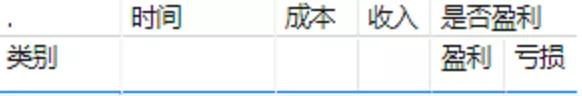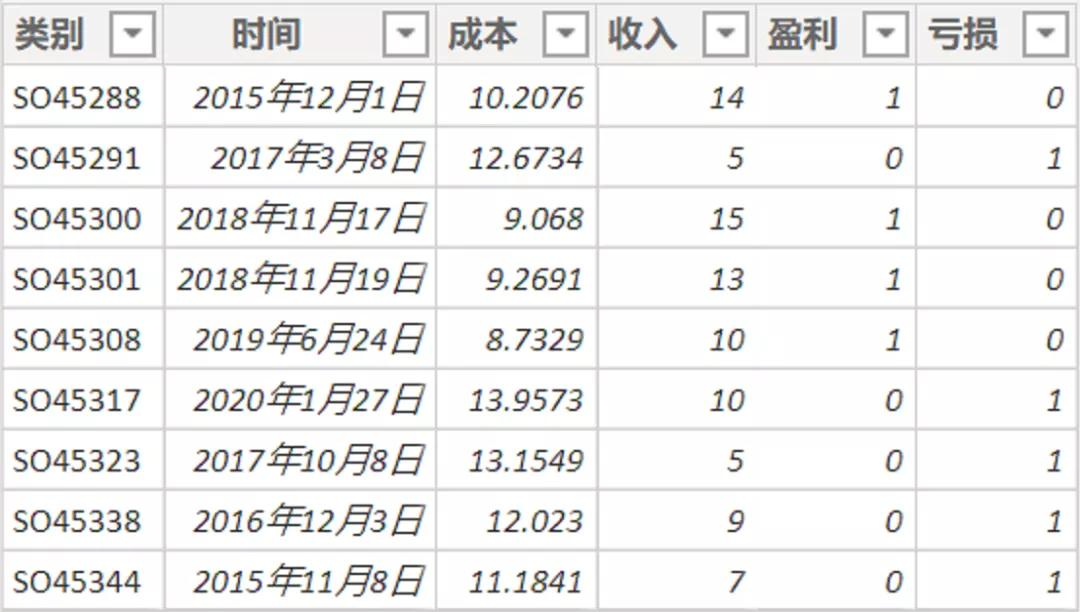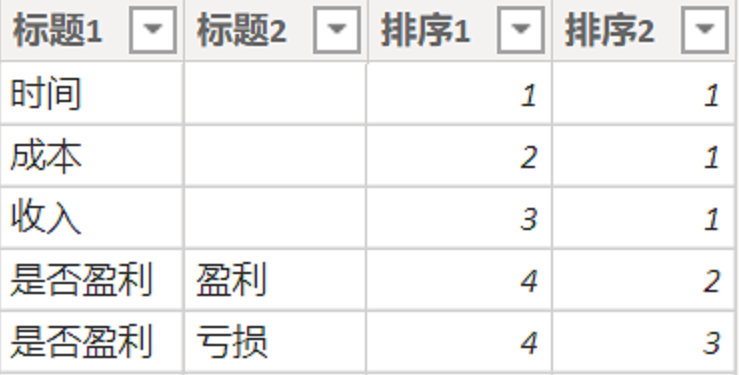IF(

SELECTEDVALUE( '表头'[标题1]) = "时间" ,

MAX('表'[时间]),

IF(

SELECTEDVALUE( '表头'[标题1]) = "成本" ,

SUM('表'[成本]),

IF(

SELECTEDVALUE( '表头'[标题1] ) = "收入" ,

SUM('表'[收入]),

IF(

SELECTEDVALUE( '表头'[标题1] ) = "是否盈利" && SELECTEDVALUE( '表头'[标题2 ] ) = "盈利" ,

IF(

SELECTEDVALUE('表'[盈利])=0,

"-",

"√"

),

IF(

SELECTEDVALUE( '表头'[标题1] ) = "是否盈利" && SELECTEDVALUE( '表头'[标题2 ] ) = "亏损" ,

IF(

SELECTEDVALUE('表'[亏损])=0,

"-",

"√"

)

)))))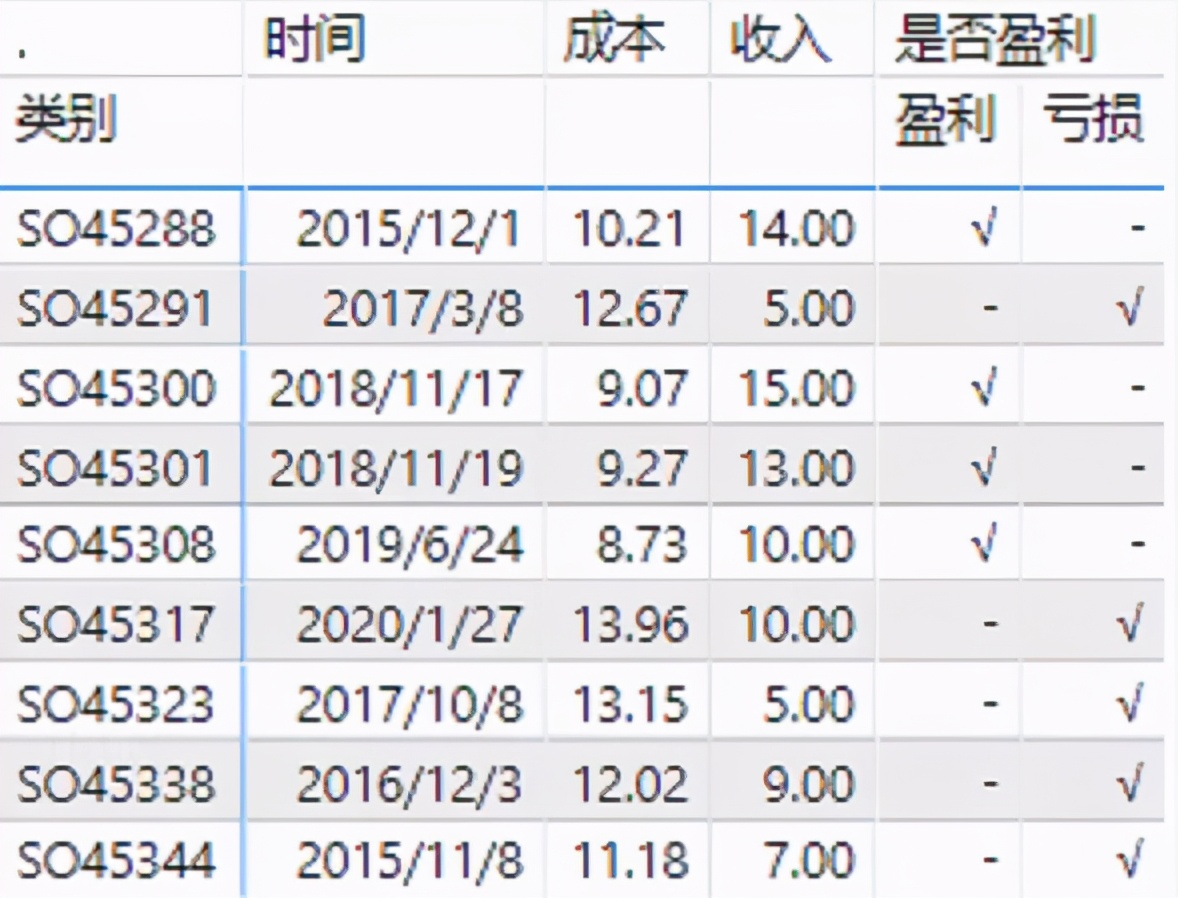FORMAT函数，这是个转换函数，官方的定义是这样的:

FORMAT
FORMAT(, )

IF(

SELECTEDVALUE( '表头'[标题1]) = "时间" ,

FORMAT(MAX('表'[时间]),"YYYY年mm月")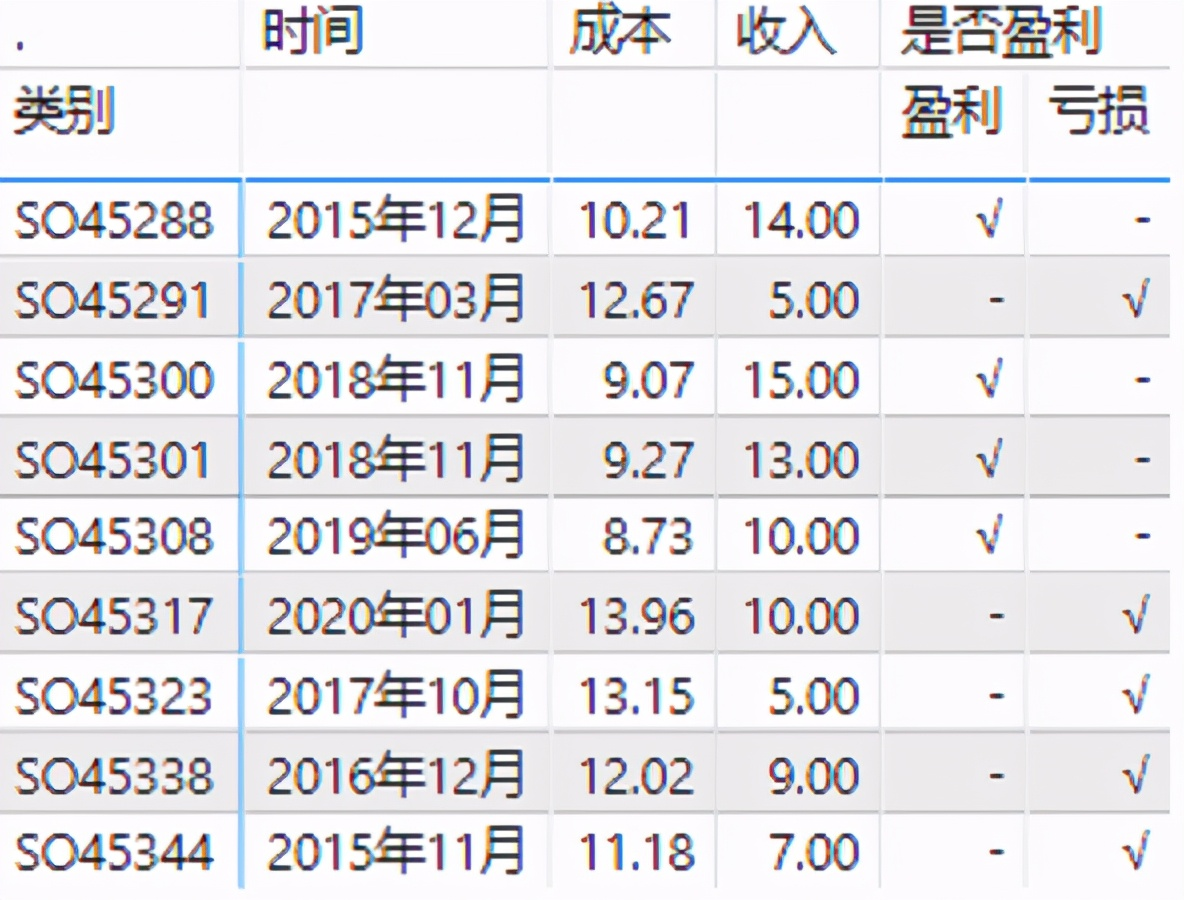IF(

SELECTEDVALUE( '表头'[标题1]) = "成本" ,

FORMAT(SUM('表'[成本]) ,"standard")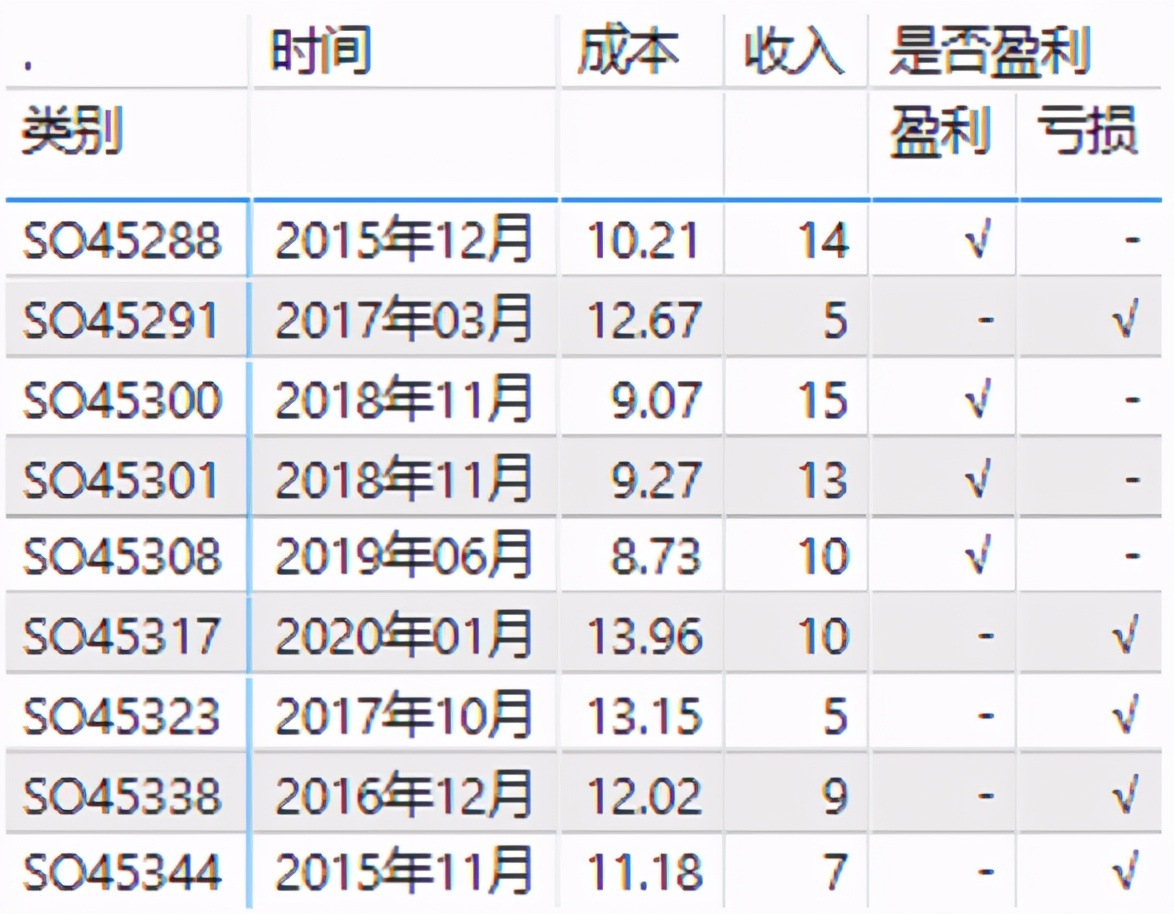https://docs.microsoft.com/zh-cn/dax/format-function-daxPower Pivot工坊